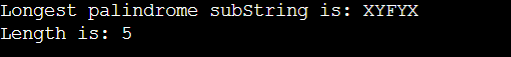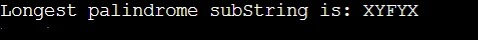# Longest Palindromic Substring

## Introduction:

We are given a String and we need to find the length of the Longest Palindromic Substring.

Let us see a few examples:

Input:

“ZXYFYXAC”

Output:

5

Explanation:

“XYFYX” is the longest palindromic substring of length 5.

Input:

“ABBBBA”

Output:

6

Explanation:

“ABBBBA” is the longest palindromic substring of length 6.

Input:

“XYZA”

Output:

0

Explanation:

There is no palindromic substring of the input String.

## APPROACH 1:

We can generate all the substrings of the input String and return the longest palindromic substring among them. We will run two for loops which will tell us the leftmost and rightmost position of the current substring. There will be another inner loop to check if the current substring is a palindrome or not.

Refer to the below implementation of the above approach.

``````import java.util.*;

class Main{

// This function prints the subString str[low..high]
static void printSubStr(String str, int low, int high)
{
for (int i = low; i <= high; ++i){
System.out.print(str.charAt(i));
}
}

/*
This function prints the
longest palindrome subString
It also returns the length
of the longest palindrome
*/
static int longestPalSubstr(String str)
{
// Get length of input String
int n = str.length();

/*
All subStrings of length 1
are palindromes
*/
int maxLength = 1, start = 0;

// Nested loop to mark start and end index
for (int i = 0; i < str.length(); i++) {
for (int j = i; j < str.length(); j++) {
int flag = 1;

// Check palindrome
for (int k = 0; k < (j - i + 1) / 2; k++){
if (str.charAt(i + k) != str.charAt(j - k)){
flag = 0;
}
}

// Palindrome
if (flag != 0 && (j - i + 1) > maxLength) {
start = i;
maxLength = j - i + 1;
}
}
}

System.out.print("Longest palindrome subString is: ");
printSubStr(str, start, start + maxLength - 1);

// return length of LPS
return maxLength;
}

// Driver Code
public static void main(String[] args)
{
String str = "ZXYFYXAC";
System.out.print("\nLength is: "+longestPalSubstr(str));
}
}
``````

Output:Time Complexity: The time complexity for the above approach is O(N * N * N) because we are running 3 nested for loops in the above approach.

Space Complexity: The space complexity for the above code is O(1) because we are using constant space for this approach.

## APPROACH 2:

We can use the Dynamic Programming approach to solve the longest palindromic substring problem.

We will make a 2D D.P. table and we will store the answer for all (i, j) pairs so that we don’t need to compute the answer for the already computed (i, j) pairs. Dp[i][j] will be 0 if the substring(i, j) is not palindrome, else it will contain its length. We will first consider all the 1 sized substrings then using the result of the 1 sized substrings we will compute the answer for the 2 sized substrings and similarly till the n sized substring.

``````import java.util.*;

class Main {

public static String longestPalSubstr(String S) {
char s[] = S.toCharArray();
int n = s.length;

//Creating a D.P. table
int dp[][] = new int[n][n];

int max = 0, x = 0, y = 0;

for(int g = 0; g < n; g++){
for(int i = 0, j = i + g; j < n; j++, i++){

if(g == 0) {
dp[i][j] = 1;
}

else if(g == 1){

/*
If s[i] == s[j] then only
the substring s(i, j) is palindrome.
*/
if(s[i] == s[j]) dp[i][j] = 2;
}
else{

/*
Current substring S(i, j) is
palindrome only if s[i] == s[j] and
the substring s(i+1, j-1) is palindrome.
*/
if(s[i] == s[j] && dp[i + 1][j - 1] != 0){
dp[i][j] = 2 + dp[i + 1][j - 1];
}
}

/*
If the Substring s(i, j) is the
longest palindromic substring
update the value of max
*/
if(dp[i][j] > max){
max = dp[i][j];
x = i;
y = j;
}
}
}

//Forming the longest palindromic substring
StringBuilder ret = new StringBuilder();
for(int i = x; i <= y; i++) {
ret.append(s[i] + "");
}

return ret.toString();
}
public static void main (String[] args) {
String str = "ZXYFYXAC";
System.out.print("Longest palindromic substring is: "+longestPalSubstr(str));
}
}
``````

Output:Time Complexity: The time complexity for the above code is O(N * N) because we are running 2 nested for loops of O(N).

Space Complexity: The space complexity for the above code is O(N * N) because we are maintaining a 2D D.P. table.

## APPROACH 3:

We can optimize Approach 2 for space complexity as follows:-

1. The idea is to generate all the even length and the odd length palindromes and keep the track of the longest palindromic substring seen so far.
2. To generate the odd length palindrome, Fix a center and expand in both directions for longer palindromes, i.e. fix the i (index) pointer as the center and two indices, i1 = i + 1 and i2 = i - 1
3. Compare i1 and i2. If they are equal then decrease the i2 pointer and increase the i1 pointer and find the maximum length.
4. Use a similar technique to find the even-length palindromic substring.
5. Take the two indices i1 = i and i2 = i - 1 and compare the characters at the i1 and i2 index and find the maximum length till all pairs of compared characters are equal and store the maximum length.
6. Return the maximum length.

``````public class Solution {
private int lo, maxLen;

public String longestPalindrome(String s) {
int len = s.length();
if (len < 2){
return s;
}

for (int i = 0; i < len-1; i++) {

//assume odd length, try to extend Palindrome as possible
extendPalindrome(s, i, i);

//assume even length.
extendPalindrome(s, i, i+1);
}
return s.substring(lo, lo + maxLen);
}

private void extendPalindrome(String s, int j, int k) {
while (j >= 0 && k < s.length() && s.charAt(j) == s.charAt(k))
{
j--;
k++;
}

if (maxLen < k - j - 1) {
lo = j + 1;
maxLen = k - j - 1;
}
}
}``````

Output:Time Complexity: The time complexity for the above code is O(N * N) because we are fixing the middle position ‘i’ and then calling extendPalindrome() function which runs in o(N) time.

Space Complexity: The space complexity for the above code is O(1) because we are using constant auxiliary space

## Approach 4:

You can even solve this question in Linear Time Complexity(O(N)). There is one pre-designed algorithm, Manacher's Algorithm which can solve this problem in O(N) time. You can learn more about this algorithm here.

However, it is a non-trivial algorithm, and it’s very difficult to come up with that algorithm in a coding interview.

## FAQs:

1. What optimization did we do on the recursive approach for the Longest Palindromic Substring problem?

• Since we were computing the answer for the same state at different times, we made a DP table to store the answer for the states we already computed, so that if in the future we need the answer for the same state we can directly return the answer from the DP table. Further, we saw how we can optimize it more so that our space complexity gets reduced to O(1).

2. What is the time complexity for the optimized approach?

• The time complexity for the optimized approach-2 is O(N * N) because we are storing the answer for all possible ‘i’ and ‘j’ pairs, and if we have already calculated the answer for any recursion call we return its answer stores in the DP table. And in approach-3 again we are taking O(N * N) time only.

## Key Takeaways:

In this blog we have covered the following things:

1. We first discussed the Recursive approach to solve this problem.
2. Then we saw how we optimized the approach by memoizing using the DP table.
3. In the end, we saw how we can optimize it more so that our space complexity gets reduced to O(1).

If you want to learn more about Dynamic Programming and want to practice some questions which require you to take your basic knowledge on Dynamic Programming a notch higher, then you can visit our Guided Path for Dynamic Programming. To practice this problem you can visit Practice Problem.

Until then, All the best for your future endeavors, and Keep Coding.